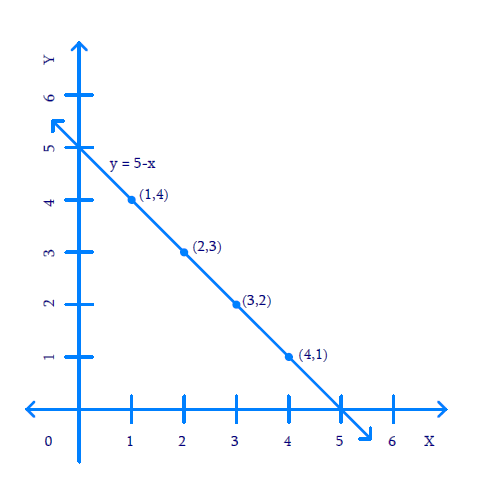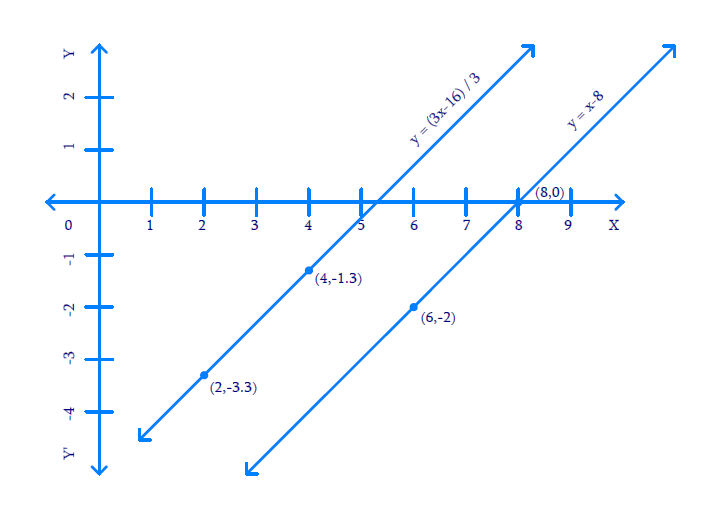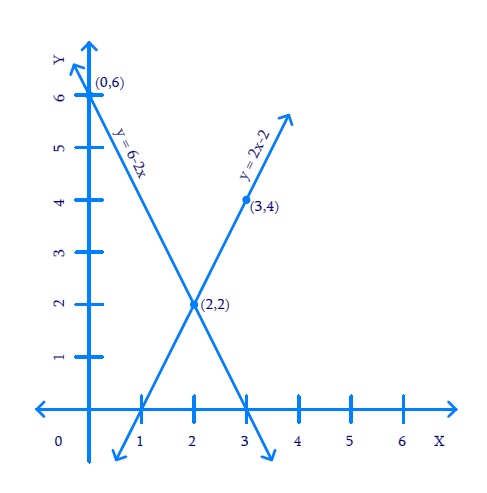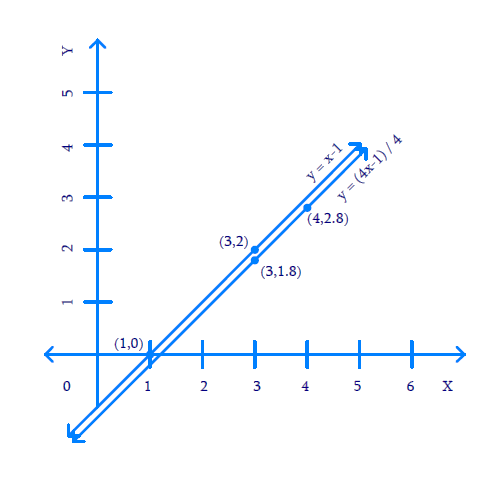# Ex.3.2 Q4 Pair of Linear Equations in Two Variables Solution - NCERT Maths Class 10

Go back to  'Ex.3.2'

## Question

Which of the following pairs of linear equations are consistent / inconsistent? If consistent, obtain the Solution graphically:

(i)

$$x + y = 5, \;2x + 2y = 10$$

(ii)

$$x-y = 8, \;3x-3y = 16$$

(iii)

$$2x + y-6 = 0, \;4x-2y-4 = 0$$

(iv)

$$2x-2y-2 = 0, \;4x-4y-5 = 0$$

Video Solution
Pair Of Linear Equations In Two Variables
Ex 3.2 | Question 4

## Text Solution

What is Unknown?

Whether the linear equations are consistent or inconsistent and graphical solution, if consistent.

Reasoning:

Consistent means pair of linear equations have one solution or infinitely many solutions.

\begin{align}{a_1}x + {b_1}y + {c_1} &= 0\\{a_2}x + {b_2}y + {c_2} &= 0\end{align}

\begin{align}&\frac{{{a_1}}}{{{a_2}}} \ne \frac{{{b_1}}}{{{b_2}}}\\& \begin{bmatrix} {\text{Intersecting lines/one}}\\ {\text{Solution}} \end{bmatrix} \\&\,\frac{{{a_1}}}{{{a_2}}} = \frac{{{b_1}}}{{{b_2}}} = \frac{{{c_1}}}{{{c_2}}}\\&\left[ \begin{array} \ {\text{Coincident Lines/Infinitely}}\\{\text{ many Solutions}} \end{array}\right]\end{align}

Inconsistent means, the lines may be parallel and do not have any Solution)

$$\frac{{{a_1}}}{{{a_2}}} = \frac{{{b_1}}}{{{b_2}}} \ne \,\,\frac{{{c_1}}}{{{c_2}}}$$ (Parallel lines/ No Solution)

(i) What is Known?

\begin{align}x + y-5 &= 0\\2x + 2y-10 &= 0\end{align}

Steps:

\begin{align}\frac{{{a_1}}}{{{a_2}}} &= \frac{1}{2}\\\frac{{{b_1}}}{{{b_2}}} &= \frac{1}{2}\\\frac{{{c_1}}}{{{c_2}}} &= \frac{{ - 5}}{{ - 10}} \\&= \frac{1}{2}\end{align}

From above

$\frac{{{a_1}}}{{{a_2}}} = \frac{{{b_1}}}{{{b_2}}} = \,\,\frac{{{c_1}}}{{{c_2}}}$

Therefore, lines are coincident and have infinitely many solutions.

Hence, they are consistent.

\begin{align}x + y-5 &= 0\\y &= - x + 5\\y& = 5-x\end{align}

 $$x$$ $$1$$ $$2$$ $$y = 5-x$$ $$4$$ $$3$$

\begin{align}2x + 2y-10 &= 0\\2y &= 10-2x\\y &= 5-x\end{align}

 $$x$$ $$3$$ $$4$$ $$y = 5-x$$ $$2$$ $$1$$All the points on coincident line are solutions for the given pair of equations.

(ii) What is Known?

\begin{align}x-y-8 &= 0\\3x-3y-16 &= 0\end{align}

Steps:

\begin{align}\frac{{{a_1}}}{{{a_2}}} &= \frac{1}{3}\\\frac{{{b_1}}}{{{b_2}}} &= \frac{{ - 1}}{{ - 3}}\\&= \frac{1}{3}\\\frac{{{c_1}}}{{{c_2}}} &= \frac{{ - 8}}{{ - 16}} \\&= \frac{1}{2}\end{align}

From above

$\frac{{{a_1}}}{{{a_2}}} = \frac{{{b_1}}}{{{b_2}}} \ne \,\,\frac{{{c_1}}}{{{c_2}}}\,$

Therefore, lines are parallel and have no solution,

Hence, the pair of equations are inconsistent.

\begin{align}x - y - 8 &= 0\\y &= x - 8\end{align}

 $$x$$ $$8$$ $$6$$ $$y{\rm{ }} = {\rm{ }}x{\rm{ }}-{\rm{ }}8$$ $$0$$ $$- 2$$

\begin{align}3x - 3y - 16 &= 0\\3y &= 3x - 16\\y &= \frac{{3x - 16}}{3}\end{align}

 $$x$$ $$2$$ $$4$$ $$y = \frac{{3x - 16}}{3}$$ $$- 3.3$$ $$- 1.3$$(iii) What is Known?

\begin{align}2x + y-6 &= 0\\4x-2y-4 &= 0\end{align}

Steps:

\begin{align}\frac{{{a_1}}}{{{a_2}}} &= \frac{2}{4}\\& = \frac{1}{2}\\\frac{{{b_1}}}{{{b_2}}} &= \frac{1}{{ - 2}} \\&= - \frac{1}{2}\\\frac{{{c_1}}}{{{c_2}}} &= \frac{{ - 6}}{{ - 4}} \\&= \frac{3}{2}\end{align}

From above:

$\frac{{{a_1}}}{{{a_2}}} \ne \frac{{{b_1}}}{{{b_2}}}\,$

Therefore, lines are intersecting and have one solution.

Hence, they are consistent.

\begin{align}2x + y-6 &= 0\\y &= 6-2x\end{align}

 $$x$$ $$0$$ $$2$$ $$y = 6-2x$$ $$6$$ $$2$$

\begin{align}4x - 2y - 4 &= 0\\2y &= 4x - 4\\y& = 2x - 2\end{align}

 $$x$$ $$2$$ $$3$$ $$y = 2x - 2$$ $$2$$ $$4$$$$x=2$$ and $$y=2$$ are solutions for the given pair of equations.

(iv) What is Known?

\begin{align}2x-2y-2 &= 0\\4x-4y-5& = 0 \\\end{align}

Steps:

\begin{align}\frac{{{a_1}}}{{{a_2}}} &= \frac{2}{4} \\&= \frac{1}{2}\\\frac{{{b_1}}}{{{b_2}}} &= \frac{{ - 2}}{{ - 4}}\\&= \frac{1}{2}\\\frac{{{c_1}}}{{{c_2}}} &= \frac{{ - 2}}{{ - 5}}\\& = \frac{2}{5}\end{align}

From above:

$\frac{{{a_1}}}{{{a_2}}} = \frac{{{b_1}}}{{{b_2}}} \ne \,\,\frac{{{c_1}}}{{{c_2}}}\,$

Therefore, lines are parallel and have no solution,

Hence, the pair of equations are inconsistent.

\begin{align}2x - 2y - 2 &= 0\\2y &= 2x - 2\\y &= x - 1\end{align}

 $$x$$ $$1$$ $$3$$ $$y = x-1$$ $$0$$ $$2$$

\begin{align}4x - 4y - 5 &= 0\\4y &= 4x - 5\\y& = \frac{{4x - 5}}{4}\end{align}

 $$x$$ $$4$$ $$3$$ $$y = \frac{{4x - 5}}{4}$$ $$2.8$$ $$1.8$$Learn from the best math teachers and top your exams

• Live one on one classroom and doubt clearing
• Practice worksheets in and after class for conceptual clarity
• Personalized curriculum to keep up with school